# Machine Learning for Software Engineers — Part 2: GoLearn

This is part two of a two-part comprehensive, high-level exploration of Machine Learning for software engineers. If you have not read Part 1 click here.

Now that we have a decent understanding of how Machine Learning works and what it is used for, it is a good time to jump into some implementation. Below are some examples that any software engineer can use to improve their project without a complex deep understanding of the inner-workings of a deep neural network.

These examples are written in Go using the GoLearn library. It is very similar to the sci-kit learn library in Python, and all examples can be translated across languages if you desire.

Linear Regression

In high school we were all at some point given a graph with points on it and told to draw a line that goes through the middle of the data. This line was called the “line of best fit.” What they didn’t tell you is that you were really doing a statistical analysis of the data.

Linear regression employs supervised learning. A set of many data points is provided to a model. These data points may have many features (x1, x2, x3), but usually only two labels (y1, y2).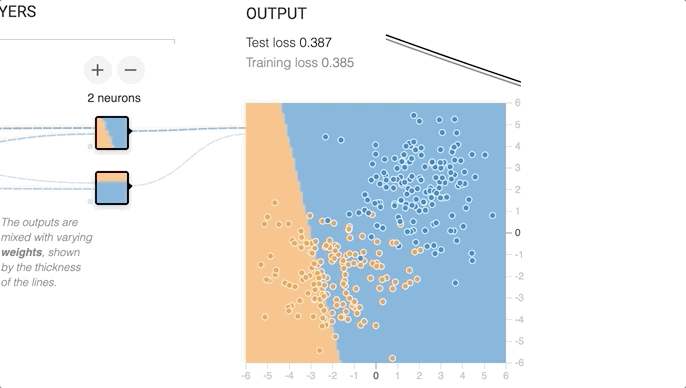In linear regression an initial random line is drawn them the model iterates through a series on new line locations until the best line is found.

The model used these features and labels to calculate a line (y=wx+b). w is the weight that is applied to each feature and b is the bias or any initial condition for the line.

While training a model, the model’s `Fit()` function picks a random bias and weights that are used to calculate the MSE. The bias and weights are then changed to get the smallest MSE possible.

There are many other variations on linear regression. There is multi-linear regression that uses many lines to classify into more than two groups. There are also quadratic regression, cubic regression, and logistic regression, where other line definitions are used. In logistic regression the line is defined as y= 1/(1 + e^(-wx)) + b. Again

weights and bias are used to optimize the line while the `Fit()`function calculates an error.

Here is an example of a linear regression program written in Go using the GoLearn package.

The first step is to bring in the data. The data object here is a matrix implementation where each column corresponds to each feature in the CSV.

`data, err := base.ParseCSVToInstances("../datasets/Advertising.csv", true) if err != nil {  panic(err) }`

Next, the data is split into training and test sets that are used to fit the model and to test the resulting model. The two parameters provided are the matrix that we want to split and the percentage that is to be in the training set. In this example, 70% is used for training and 30% is used for testing. It is important to note that this function does randomize the data as it splits between training and test sets. This is to prevent bias that may be included in the ordering of the data in the csv.

`trainData, testData := base.InstancesTrainTestSplit(data, 0.70)`

Now to fit and test the model.

`r := regression.NewLinearRegression() err = r.Fit(trainData) if err != nil {  panic(err) } predictions, err := r.Predict(testData) if err != nil {  panic(err) }`

Lastly, view the results of the fit.

`fmt.Println("Linear Regression (information gain)") cf, err := evaluation.GetConfusionMatrix(testData, predictions) if err != nil {  panic(fmt.Errorf("Unable to get confusion matrix: %s", err.Error())) } fmt.Println(evaluation.GetSummary(cf)) }`

Decision Tree

Another commonly used model is the decision tree. The decision tree uses a series of nodes and branches to make selections across each of the data’s features. These trees can have hundreds of nodes and handle complex models with hundreds of features.

The following example will be an end to end implementation of a name classification model. It used a data set of tweets about the 2018 FIFA World Cup downloaded from Kaggle.

First thing is to get the data from the CSV. The data is stored into a slice and struct that will be used to get the appropriate features for training and testing the model.

`// Tweet object for holding raw form of csv data.type Tweet struct { ID            int Lang          string Date          int64 Source        string Length        int OrgTweet      string Tweets        string Likes         int Retweets      int Hashtag       string Usermention   string UserMentionID string Name          string Place         string Followers     int Friends       int}func getData(file string) ([]Tweet, error) { f, err := os.Open(file) defer f.Close() if err != nil {  return nil, err } lines, err := csv.NewReader(f).ReadAll() if err != nil {  return nil, err } var tweets []Tweet for i, line := range lines {  if i == 0 {   continue  }  id, _ := strconv.Atoi(line)  layout := "2006-01-02"  d, _ := time.Parse(layout, line)  l, _ := strconv.Atoi(line)  likes, _ := strconv.Atoi(line)  r, _ := strconv.Atoi(line)  f, _ := strconv.Atoi(line)  friends, _ := strconv.Atoi(line)   t := Tweet{   ID:            id,   Lang:          line,   Date:          d.Unix(),   Source:        line,   Length:        l,   OrgTweet:      line,   Tweets:        line,   Likes:         likes,   Retweets:      r,  Hashtag:       line,   Usermention:   line,   UserMentionID: line,   Name:          line,   Place:         line,   Followers:     f,   Friends:       friends,  }  tweets = append(tweets, t) } return tweets, err}`

Feature engineering is the fancy name for getting the viable data for your model and cleaning it up. To classify names for other words, we need to pull out and label text features.

`func plotWords(df dataframe.DataFrame) error { var data []WordData mapCounts := make(map[string]int, 100000)  // make map of names that will be labeled as true  // there are two columns that contain name data. WE need to take names from // both columns colNames := []string{"Name", "Usermention"} for _, colName := range colNames {  vals := df.Col(colName).Records()  for _, val := range vals {   // split multi word names into single words   words := strings.Split(val, " ")   for _, w := range words {    // remove spaces    w = strings.Trim(w, " ")    if _, ok := mapCounts[w]; !ok {     mapCounts[w] = 1     continue    }    mapCounts[w]++   }  }  // add data to struct that contains important data information.  isName := true  for name, count := range mapCounts {   d := WordData{    Word:       name,    Occurances: count,    IsName:     isName,   }   data = append(data, d)  } } // parse each word in the tweets and lable it as name or not records := df.Col("OrgTweet").Records() tweetWordsCount := make(map[string]int, 100000) for _, r := range records {  words := strings.Split(r, " ")  for _, w := range words {   w = strings.Trim(w, " ")   // skip names that we already have   if mapCounts[w] > 0 {    continue   }   // we assume we have all the names. This is going   // cause some un realiability in the data.   if _, ok := tweetWordsCount[w]; !ok {    tweetWordsCount[w] = 1    continue  }   tweetWordsCount[w]++  } } // add stata to struct for name, count := range tweetWordsCount {  d := WordData{   Word:       name,   Occurances: count,   IsName:     false,  }  data = append(data, d) } fmt.Printf("From the Fifa World Cup Data you have parsed out %d unique words. \n", len(data))  // create a new data frame newDF := dataframe.LoadStructs(data) fmt.Println(newDF) // get summary of word occurances fmt.Println(newDF.Select([]string{"Occurances"}).Describe()) // cache data frame in csv myFile, err := os.Create("data/words.csv") if err != nil {  return err } err = newDF.WriteCSV(myFile) if err != nil {  return err } return nil}`

Just like before, we have to import data, train test split, define the model, Fit, Predict, and print the results.

`// DecisionTree Write and train DecisionTree model.func DecisionTree(file string) error { // var wg sync.WaitGroup rand.Seed(44111342)  // Load in the words dataset words, err := base.ParseCSVToInstances(file, true) if err != nil {  return err } // Create a 70-30 training-test split trainData, testData := base.InstancesTrainTestSplit(words, 0.70) fmt.Println("ID3Decision Tree train") tree := trees.NewID3DecisionTree(0.6) // Train the ID3 tree err = tree.Fit(trainData) if err != nil {  return err } // Generate predictions predictions, err := tree.Predict(testData)if err != nil {  return err } // Evaluate fmt.Println("ID3 Performance (information gain)") cf, err := evaluation.GetConfusionMatrix(testData, predictions) if err != nil {  return fmt.Errorf("Unable to get confusion matrix: %s", err.Error()) } fmt.Println(evaluation.GetSummary(cf)) tree.Save("models/DecisonTree.h") return err}`

If you are brave you can go ahead, visit the GoLearn, and start implementing you own model.

--

--

--

## More from Weave Lab

Lessons learned from the Weave development lab

## Statistical Learning (V): Unsupervised Learning## 63 Tensorflow Courses on Coursera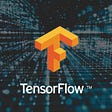## A review of Generative Adversarial Networks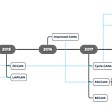## Analyzing Airbnb market in NYC (using R)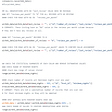## Ghana NLP — Computational Mapping of Ghanaian Languages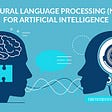## Review of Deep Learning Architectures for Image Classification Problem (Part 3)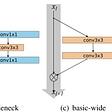## A Deep Dive Into The Concept of Regression## Miriah Peterson

Data Engineer | ML hobbiest | Golang Evangelist | Presenter

## Golang 1.18 — Generics explained## How Golang DB migration tool work?## Go Diagnostics 101## Golang Field Alignment